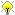[Documentation] [TitleIndex] [WordIndex]

 Note: This tutorial assumes that you have completed the previous tutorials: Building a Visual Robot Model with URDF from Scratch, Building a Movable Robot Model with URDF.Please ask about problems and questions regarding this tutorial on answers.ros.org. Don't forget to include in your question the link to this page, the versions of your OS & ROS, and also add appropriate tags.

# Adding Physical and Collision Properties to a URDF Model

Description: Learn how to add collision and inertial properties to links, and how to add joint dynamics to joints.

Keywords: URDF, Inertial, Dynamics, Collision

Tutorial Level: BEGINNER

Next Tutorial: Using Xacro to Clean Up a URDF File Using a URDF in Gazebo

In this tutorial, we’ll look at how to add some basic physical properties to your URDF model and how to specify its collision properties.

## Collision

So far, we’ve only specified our links with a single sub-element, visual, which defines (not surprisingly) what the robot looks like. However, in order to get collision detection to work or to simulate the robot in something like Gazebo, we need to define a collision element as well. Here is the new urdf with collision and physical properties.

Here is the code for our new base link.

```   1 <link name="base_link">
2     <visual>
3       <geometry>
5       </geometry>
6       <material name="blue">
7         <color rgba="0 0 .8 1"/>
8       </material>
9     </visual>
10     <collision>
11       <geometry>
13       </geometry>
14     </collision>
```
• The collision element is a direct subelement of the link object, at the same level as the visual tag
• The collision element defines its shape the same way the visual element does, with a geometry tag. The format for the geometry tag is exactly the same here as with the visual.
• You can also specify an origin in the same way as a subelement of the collision tag (as with the visual)

In many cases, you’ll want the collision geometry and origin to be exactly the same as the visual geometry and origin. However, there are two main cases where you wouldn’t.

• Quicker Processing - Doing collision detection for two meshes is a lot more computational complex than for two simple geometries. Hence, you may want to replace the meshes with simpler geometries in the collision element.
• Safe Zones - You may want to restrict movement close to sensitive equipment. For instance, if we didn’t want anything to collide with R2D2’s head, we might define the collision geometry to be a cylinder encasing his head to prevent anything from getting to near his head.

## Physical Properties

In order to get your model to simulate properly, you need to define several physical properties of your robot, i.e. the properties that a physics engine like Gazebo would need.

### Inertia

Every link element being simulated needs an inertial tag. Here is a simple one.

```   1   <link name="base_link">
2     <visual>
3       <geometry>
5       </geometry>
6       <material name="blue">
7         <color rgba="0 0 .8 1"/>
8       </material>
9     </visual>
10     <collision>
11       <geometry>
13       </geometry>
14     </collision>
15     <inertial>
16       <mass value="10"/>
17       <inertia ixx="0.4" ixy="0.0" ixz="0.0" iyy="0.4" iyz="0.0" izz="0.2"/>
18     </inertial>
```
• This element is also a subelement of the link object.
• The mass is defined in kilograms.
• The 3x3 rotational inertia matrix is specified with the inertia element. Since this is symmetrical, it can be represented by only 6 elements, as such.
 ixx ixy ixz ixy iyy iyz ixz iyz izz
• This information can be provided to you by modeling programs such as MeshLab. The inertia of geometric primitives (cylinder, box, sphere) can be computed using Wikipedia's list of moment of inertia tensors (and is used in the above example).

• The inertia tensor depends on both the mass and the distribution of mass of the object. A good first approximation is to assume equal distribution of mass in the volume of the object and compute the inertia tensor based on the object's shape, as outlined above.
• If unsure what to put, a matrix with ixx/iyy/izz=1e-3 or smaller is often a reasonable default for a mid-sized link (it corresponds to a box of 0.1 m side length with a mass of 0.6 kg). Although often chosen, the identity matrix is a particularly bad default, since it is often much too high (it corresponds to a box of 0.1 m side length with a mass of 600 kg!).
• You can also specify an origin tag to specify the center of gravity and the inertial reference frame (relative to the link's reference frame).
• When using realtime controllers, inertia elements of zero (or almost zero) can cause the robot model to collapse without warning, and all links will appear with their origins coinciding with the world origin.

### Contact Coefficients

You can also define how the links behave when they are in contact with one another. This is done with a subelement of the collision tag called contact_coefficients. There are three attributes to specify:

### Joint Dynamics

How the joint moves is defined by the dynamics tag for the joint. There are two attributes here:

• friction - The physical static friction. For prismatic joints, the units are Newtons. For revolving joints, the units are Newton meters.
• damping - The physical damping value. For prismatic joints, the units are Newton seconds per meter. For revolving joints, Newton meter secons per radian.

If not specified, these coefficients default to zero.

## Other Tags

In the realm of pure URDF (i.e. excluding Gazebo-specific tags), there are two remaining tags to help define the joints: calibration and safety controller. Check out the spec, as they are not included in this tutorial.

## Next Steps

Reduce the amount of code and annoying math you have to do by using xacro.

2020-01-11 13:14